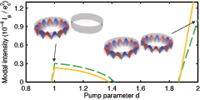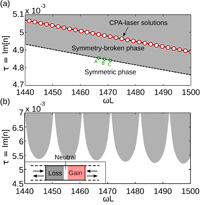## Exceptional points and lasing self-termination in photonic molecules

Ramy El-Ganainy, Mercedeh Khajavikhan, Li Ge

We investigate the rich physics of photonic molecule lasers using a non-Hermitian dimer model. We show that several interesting features, predicted recently using a rigorous steady state ab-initio laser theory (SALT), can be captured by this toy model. In particular, we demonstrate the central role played by exceptional points in both pump-selective lasing and laser self-terminations phenomena. Due to its transparent mathematical structure, our model provides a lucid understanding for how different physical parameters (optical loss, modal coupling between microcavities and pump profiles) affect the lasing action. Interestingly, our analysis also confirms that, for frequency mismatched cavities, operation in the proximity of exceptional points (without actually crossing the square root singularities) can still lead to laser self-termination. We confirm this latter prediction for two coupled slab cavities using scattering matrix analysis and SALT technique. In addition, we employ our model to investigate the pump-controlled lasing action and we show that emission patterns are governed by the locations of exceptional points in the gain parameter space. Finally we extend these results to multi-cavity photonic molecules, where we found the existence of higher-order EPs and pump-induced localization.

http://arxiv.org/abs/1404.1242

Optics (physics.optics)

## Parity-Time Symmetry Breaking beyond One Dimension: the Role of Degeneracy

Li Ge, A. Douglas Stone

We consider the role of degeneracy in Parity-Time (PT) symmetry breaking for non-hermitian wave equations beyond one dimension. We show that if the spectrum is degenerate in the absence of T-breaking, and T is broken in a generic manner (without preserving other discrete symmetries), then the standard PT-symmetry breaking transition does not occur, meaning that the spectrum is complex even for infinitesimal strength of gain and loss. However the reality of the entire spectrum can be preserved over a finite interval if additional discrete symmetries X are imposed when T is broken, if X decouple all degenerate modes. When this is true only for a subset of the degenerate spectrum, there can be a partial PT transition in which this subset remains real over a finite interval of T-breaking. If the spectrum has odd-degeneracy, a fraction of the degenerate spectrum can remain in the symmetric phase even without imposing additional discrete symmetries, and they are analogous to dark states in atomic physics. These results are illustrated by the example of different T-breaking perturbations of a uniform dielectric disk and sphere. Finally, we show that multimode coupling is capable of restoring the PT-symmetric phase at finite T-breaking. We also analyze these questions when the parity operator is replaced by another spatial symmetry operator and find that the behavior can be qualitatively different.

http://arxiv.org/abs/1402.0428
Quantum Physics (quant-ph); Optics (physics.optics)

## Breaking of PT-symmetry in bounded and unbounded scattering systems

Philipp Ambichl, Konstantinos G. Makris, Li Ge, Yidong Chong, A. Douglas Stone, Stefan Rotter

PT-symmetric scattering systems with balanced gain and loss can undergo a symmetry-breaking transition in which the eigenvalues of the non-unitary scattering matrix change their phase shifts from real to complex values. We relate the PT-symmetry breaking points of such an unbounded scattering system to those of underlying bounded systems. In particular, we show how the PT-thresholds in the scattering matrix of the unbounded system translate into analogous transitions in the Robin boundary conditions of the corresponding bounded systems. Based on this relation, we argue and then confirm that the PT-transitions in the scattering matrix are, under very general conditions, entirely insensitive to a variable coupling strength between the bounded region and the unbounded asymptotic region, a result which can be tested experimentally.

http://arxiv.org/abs/1307.0149

Optics (physics.optics); Quantum Physics (quant-ph)

## Antisymmetric PT-photonic structures with balanced positive and negative index materials

Li Ge, H. E. Tureci

In this Letter we study a new class of synthetic materials in which the refractive index satisfies a special symmetry, $$n(-x)=-n^*(x)$$, which we term antisymmetric parity-time (APT) systems. Unlike PT-symmetric systems which require balanced gain and loss, i.e. $$n(-x)=n^*(x)$$, APT systems consist of balanced positive and negative index materials (NIMs). Despite the seemingly PT-symmetric optical potential $$V(x)\equiv n(x)^2\omega^2/c^2$$, such systems are not invariant under combined PT operation due to the discontinuity of the spatial derivative of the wavefunction. We show that APT systems display intriguing properties such as spontaneous phase transition of the scattering matrix, bidirectional invisibility, and a continuous lasing spectrum.

http://arxiv.org/abs/1208.4644
Optics (physics.optics)

## Conservation relations and anisotropic transmission resonances in one-dimensional PT-symmetric photonic heterostructures

Li Ge, Y. D. Chong, A. D. Stone

We analyze the optical properties of one-dimensional (1D) PT-symmetric structures of arbitrary complexity. These structures violate normal unitarity (photon flux conservation) but are shown to satisfy generalized unitarity relations, which relate the elements of the scattering matrix and lead to a conservation relation in terms of the transmittance and (left and right) reflectances. One implication of this relation is that there exist anisotropic transmission resonances in PT-symmetric systems, frequencies at which there is unit transmission and zero reflection, but only for waves incident from a single side. The spatial profile of these transmission resonances is symmetric, and they can occur even at PT-symmetry breaking points. The general conservation relations can be utilized as an experimental signature of the presence of PT-symmetry and of PT-symmetry breaking transitions. The uniqueness of PT-symmetry breaking transitions of the scattering matrix is briefly discussed by comparing to the corresponding non-Hermitian Hamiltonians.

http://arxiv.org/abs/1112.5167
Optics (physics.optics)

## Pump-induced Exceptional Points in Lasers above ThresholdM. Liertzer, Li Ge, A. Cerjan, A. D. Stone, H. E. Türeci, S. Rotter

We demonstrate that the above-threshold behavior of a laser can be strongly affected by exceptional points which are induced by pumping the laser non-uniformly. At these singularities the eigenstates of the non-Hermitian operator which describes the lasing modes coalesce. In the vicinity of these points the laser may turn off even when the overall pump power deposited in the system is increased. We suggest that such signatures of a pump-induced exceptional point can be experimentally probed with coupled ridge or microdisk lasers.

http://arxiv.org/abs/1109.0454
Optics (physics.optics)

http://arxiv.org/abs/1109.0454

## PT-symmetry breaking and laser-absorber modes in optical scattering systems

Y. D. Chong, Li Ge, A. Douglas StoneUsing a scattering matrix formalism, we derive the general scattering properties of optical structures that are symmetric under a combination of parity and time-reversal (PT). We demonstrate the existence of a transition beween PT-symmetric scattering eigenstates, which are norm-preserving, and symmetry-broken pairs of eigenstates exhibiting net amplification and loss. The system proposed by Longhi, which can act simultaneously as a laser and coherent perfect absorber, occurs at discrete points in the broken symmetry phase, when a pole and zero of the S-matrix coincide.

http://arxiv.org/abs/1008.5156
Optics (physics.optics); Quantum Physics (quant-ph)